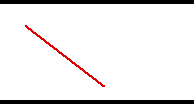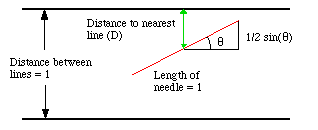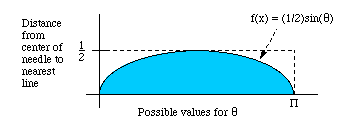College of Education

# Office for Mathematics, Science, and Technology Education

Resources Projects Contact
Home Activity Buffon

# Buffon's Needle

## Buffon's Needle An Analysis and Simulation## Introduction

Buffon's Needle is one of the oldest problems in the field of geometrical probability. It was first stated in 1777. It involves dropping a needle on a lined sheet of paper and determining the probability of the needle crossing one of the lines on the page. The remarkable result is that the probability is directly related to the value of pi.

This page will present an analytical solution to the problem along with a JavaScript applet for simulating the needle drop in the simplest case scenario in which the length of the needle is the same as the distance between the lines.

## The Simplest Case

Let's take the simple case first. In this case, the length of the needle is one unit and the distance between the lines is also one unit. There are two variables, the angle at which the needle falls (θ) and the distance from the center of the needle to the closest line (D). Theta can vary from 0 to 180 degrees and is measured against a line parallel to the lines on the paper. The distance from the center to the closest line can never be more that half the distance between the lines. The graph below depicts this situation.The needle in the picture misses the line. The needle will hit the line if the closest distance to a line (D) is less than or equal to 1/2 times the sine of theta. That is, D ≤ (1/2)sin(θ). How often will this occur?

In the graph below, we plot D along the ordinate and (1/2)sin(θ) along the abscissa. The values on or below the curve represent a hit (D ≤ (1/2)sin(θ)). Thus, the probability of a success it the ratio shaded area to the entire rectangle. What is this to value?The shaded portion is found with using the definite integral of (1/2)sin(θ) evaluated from zero to pi. The result is that the shaded portion has a value of 1. The value of the entire rectangle is (1/2)(π) or π/2. So, the probability of a hit is 1/(π/2) or 2/π. That's approximately .6366197.

To calculate pi from the needle drops, simply take the number of drops and multiply it by two, then divide by the number of hits, or 2(total drops)/(number of hits) = π (approximately).

## The Other Cases

There are two other possibilities for the relationship between the length of the needles and the distance between the lines. A good discussion of these can be found in Schroeder, 1974. The situation in which the distance between the lines is greater than the length of the needle is an extension of the above explanation and the probability of a hit is 2(L)/(K)π where L is the length of the needle and K is the distance between the lines. The situation in which the needle is longer than the distance between the lines leads to a more complicated result.

## Simulation

In this simulation, press one of the buttons labelled "Drop" to drop a batch of needles on the parallel lines. The measurements and calculations will be completed for you and displayed below the illustration. Each batch of needles you drop will add to the total number of needles measured, allowing you to approximate pi more precisely with each drop. The illustration will show the most recent batch of needles dropped.

Further down, you can also change the scale of the needles dropped or restart the experiment from the beginning. Note that if you change the needle scale, the experiment will automatically reset itself the next time you drop needles, because all the needles need to be the same size and shape for the calculations to work.

We're sorry, your browser does not support HTML5 Canvas...MeasurementValue
Needle Scale
Extent = Perimeter / Greatest Vertex Distance
Number of Drops
Number of Hits
Drops / Hits
π ≈ 2 * Extent * Scale * Drops / Hits

Drop Shape

### Simulation Credits

• Analysis and original Java applet by George Reese (1996)
• Modified by Pavel Safronov (2003-2005)
• JavaScript+HTML5 applet remake by Evan Ramos (2014-2015)

## References

Cheney, W. and Kincaid, D. (1985). Numerical Mathematics and Computing. 2nd Ed. Pace Grove, California: Brooks/Cole Publishing Company pp. 354-354

Schroeder, L. (1974). Buffon's needle problem: An exciting application of many mathematical concepts. Mathematics Teacher, 67 (2), 183-186.